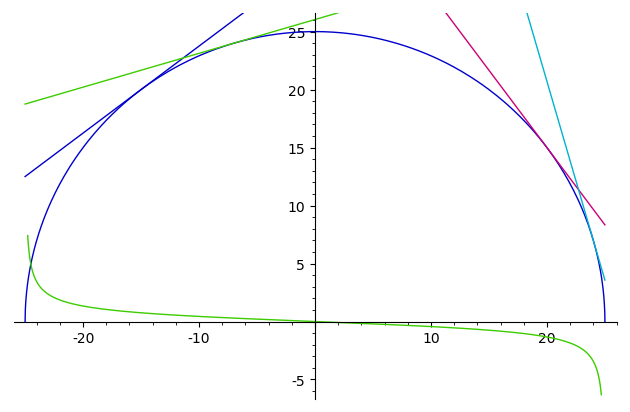# How to plot multiple tangent lines on a graph

I installed Sage today, so I am unfamiliar with the software. I have an equation f(x)=sqrt(625-x^2), and f'(x)=-x/sqrt(625-x^2). I am trying to plot both f(x) and f'(x) at 20, 24, -7 and -15 on one graph. To begin I tried:

plot(sqrt(625-x^2) + plot(-x/sqrt(625-^2))

This produced:Please let me know if the image doesn't appear as this is my first question. Essentially it is a straight line at the intercepts of 25 and 0 on the y axis. How would I create a simple graph that demonstrates f(x) and f'(x) tangent lines at x = 20, 24, -7 and -15? Many thanks.

edit retag close merge delete

I am a Quantitative Epidemiology PhD student brushing up on my calculus skills using a free textbook online called Multivariate analysis.

Sort by » oldest newest most voted

I am a Quantitative Epidemiology PhD student brushing up on my calculus skills using a free textbook online called Multivariate analysis.

Okay, a real call for help. We tend to avoid giving pre-cooked answers to lazy undergrads' homework...

f(x)=sqrt(625-x^2)
Curves=plot([f, f.diff(x)],(-25, 25), ymin=-6, ymax=26, aspect_ratio=1)
Tangents=plot([(f(u)+(x-u)*f.diff(x)(u)) for u in [-15, -7, 20, 24]], (x, -25, 25))
Curves+TangentsThis code takes advantage of a couple not-so-obvious Sage features:

• plot accepts a sequence (here, lists) as first argument.
• In the first call (to get Curves), this is a list of (symbolic) functions; the second argument is just a range for the argument of the function(s).
• In the second case, it is a list of symbolic expressions; Therefore, the second argument specifies the name of the (symbolic) variable taking values in the range it specifies.
• I didn't bother to fix the colors, the labels nor a legend; this is left as an (useful) exercise for the reader.
• I did specify a range for the ordinates in the first plot. It is also used for the superposition of the two plots.

A lot more could be said. But:

r.library("fortunes")
r.fortune("'TFM'")

This is all documented in TFM. Those who WTFM don't want to have to WTFM again
on the mailing list. RTFM.
-- Barry Rowlingson
R-help (October 2003)


But you're in luck. TFM is marvelously supplemented by this book, freely available, which will certainly help you (even if some details (notebook) have changed and others (Python 3) will change soon).

If you're not primarily a native English speaker, the original French version, or a German translation are also available via the same page.

more

PS: Would you mind sharing the references of your multivariate analysis textbook, apd possibly what you think of it ? This anwer could be of interest to many Sage` would-be and beginning users...